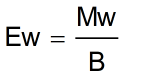Request a Tool

Weight of Acid Calculator

To calculate the estimated weight of acid

Output

Equivalent weight of acid (Ew)
0

Formula• Mw = Molecular weight of acid
• B = Basicity of acid
• Ew = Equivalent weight of acid

Defination / Uses

The basicity of an acid is the number of replaceable hydrogen atoms present in the molecule. The estimated weight of acid is determined by using Acid Weight Calculator.

The approximate weight of acid is calculated using this Acid Weight Calculator. It calculates the exact equivalent weight by using the acid's molecular weight and basicity. If you're interested in learning more about the notion, go ahead and investigate it. In a fraction of a second, use the free Equivalent Weight Calculator tool to get the equivalent weight of an acid. Lets get understand by an example:
C2H6 has a molecular mass of roughly 30 ((2 x 12) + (6 x 1)). As a result, the molecule is about 2.5 times heavier than the 12C atom, or about the same mass as the NO atom, which has a molecular mass of 30 or (14+16). Basicity of an acid is its valency. Ew symbol represents the equivalent weight of an acid. Use the upper given formula for manual calculations. No sign-up, registration OR captcha is required to use this tool.